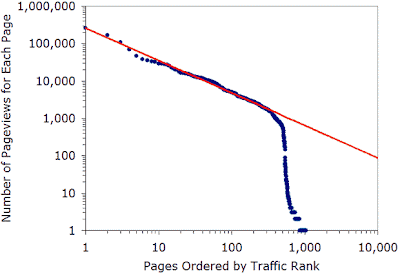### Mathematical RealityWhy do we use so much of mathematics in analytics, and is there any way to justify this method? The answer is very straight and simple. Mathematics is taken as one of the most truly logical language. No doubt.

In addition, analytics means analysing the facts and logically following the clues in the facts to come to a practical conclusion. Its this process that exploits the power of mathematics, to structure the logic in the most appropriate way. The mathematical processing makes the logic in physical world more understandable and playable. Thus, giving analyst a tremendous power to handle the facts.

If there is a time, than I would suggest going through a book called "A Mathematician's Apology" by  G.H. Hardy. It is the most humblest book I have come across. The book is about a Mathematician who have chosen to devote his whole life on mathematics. There, he argues the difference between the Mathematical Reality and Physical Reality. Let me explain it in my words.

In a physical world, there are many qualities, lets call it variables which are given specific units. All these variables are in continuous change. One of the most important variable that changes almost every instance is no other than "time". (Be careful with what I have said right now, because its a very complicated statement, as change in itself is related to time). And all these variables are dependent upon one other, making it almost impossible to track them. This fact makes it impossible to consider every single variable during our analysis. Therefore, its impossible to model any physical system with 100% accuracy.But in mathematical world, every thing is ideal. In order to understand this idealism, I want to give some examples: lets take a circle or line or any geometric shape.In real world, when a circle is drawn, its never circle and also occupies physical space. But, in mathematics, line is considered to be 100% straight and ideally of zero thickness occupying no space at all.

Further, this circle is described by an algebraic equations. This mathematical equation is like the DNA of life, describing it in all possible ways.

Therefore, in mathematical domain, every calculations and predictions are accurate as concerned with logic but when we map them to physical domain, we get errors. These errors are due to the approximations in our analysis and due to the undeniable difference between the two domain.In the graph by the side, blue line is the real data from physical world, whereas the red line is the data from the mathematical world.

If the data is considered from 1 to 100 in the X-axis, we can see that the two lines are almost following each other. By taking this sample and modeling in the mathematical domain, we come to conclusion that the physical domain must follow the red line pattern. But NO! Some how, the real world's data is dropping down. There is tremendous error. This error can be reduced with more consideration of all the variables. T

The point is, its impossible to accurately model the physical system into a mathematical system with zero percent error. Therefore, we talk about approximation, probability and percentages. There is always a loss in the process of transformation of variables, from one domain to another. Then, why do we take this step to analyse. Its because, once the problem is in mathematics, its easier to manipulate the variables and  create a logical relations, this is made more easier by the availability of thousand and thousands of mathematical tools. And off course, the problem cannot be solved in the physical domain, it has to be transformed to mathematical domain.

Understanding these relationships between the physical variable and a mathematical variable makes a lot of difference in our analysis process.  Let me know what you think about it.

### Selling a Comb to a Bald Person?

Here my friend, Ashay, put it very truly to me that the marketer's most challenge is to sell a comb to a bald. First, I am not trying to justify anything here. But I just couldn't help thinking how on earth am I going to sell a comb to a bald. How? Just how? I kept pondering upon it till late night. I actually had very few options with me, the first was obviously to use Google and Find? :) But, I didn't do that. Some how I was still in confusion. Then just before going to sleep, I had an discussion with my other friend, on types of marketing on issues related to customer centric marketing. Hmm. Then some how it hit me. I went back to basic on my own philosophy, sell things that is needed. So here is a small anecdote I prepared : Sale Person   : Hello sir. How are you? Do you have a time, plzzz? Bald Person : (Almost confused and in social causality) OK OK what is it? I don't have time. Sale Person    : Here sir, do you want to by a comb?  Bald Person  : Can'